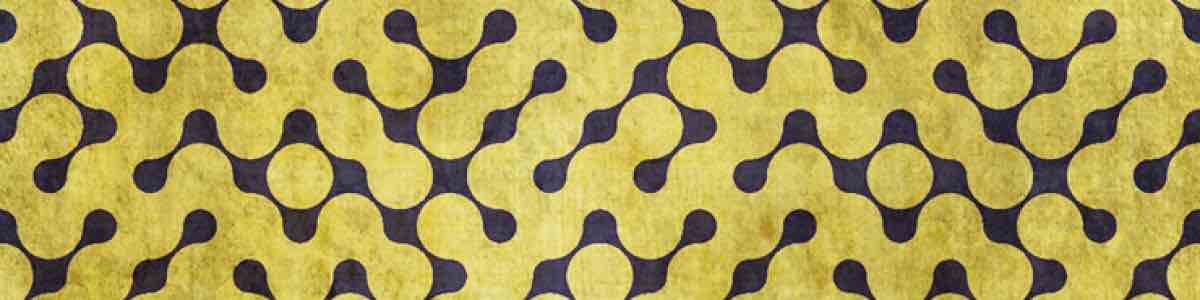Updated by lexiestevenson821 on Oct 15, 2016
REPORT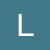lexiestevenson821
Owner
10 items   1 followers   0 votes   6 views

Volume of Rectangular Prisms

Here are some useful sources for learning about the volume of rectangular prisms.

1

Volume of a rectangular prismThis is a video from the khan academy website explaining how to solve for the area of a rectangular prism. It will help students understand the process better if they are still having trouble understanding it.

2

Practicing Fifth grade math: 'Volume of cubes and rectangular prisms'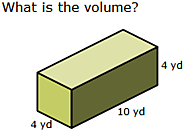This is a link to IXL which has students practice finding the volume of a rectangular prism. This will give them practice actually carrying out the process being learned.

3

MathVillage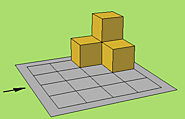This website gives students shapes and asks them to tell how many cubic units the shape is. This is a good website because it shows the students that a shape is the number of cubic units in the first level plus the number of cubic units in the next level. Students can apply that to rectangular prisms by realizing that it is the same number of units in each layer.

4

Volume of Cubes and Rectangular Prisms - Grade 5 - Practice with Math Games

This website provides more practice for students. It is a good way for the students to apply and practice the principles they are learning.

5

XP Math Games ArcadeThis website gives the students practice finding volume quickly. It is a good site to use because it uses minecraft which is something the students will be familiar with and it also forces them to increase their speed.

6

Volume Shapes Shoot Game - Geometry: Math GamesThis game makes practicing volume fun. It also helps students see that the volume of a rectangular prism is the number of cubic units in the first layer multiplied by the number of layers.

7

Volume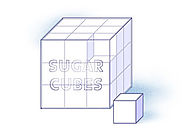This website shows students how to solve the volume of a rectangular prism by first finding the area of the bottom and then figuring out how many layers of that area will fill the prism. This is a good website to help students visualize volume better.

8

Who Wants to be a Volume Millionaire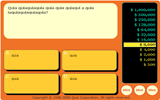This is a fun game to help students think about the volume of a rectangular prism in multiple ways. This game helps students find the volume and the length of the sides and it also forces them to think about how to label units of volume.

9

Volume of a Rectangular Prism

This website provides an opportunity for students to practice the process of finding volume while trying to beat a personal best score. I like this website because the visual is gone so it is the next step and requires students to know the formula.

10

Feliks Zemdegs retains his title with an improved time of 7.56 seconds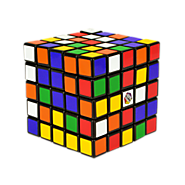I want to use a picture of a rubik's cube for my thinglink. This will show the students a real life object that is a rectangular prism and shows the cubic units.